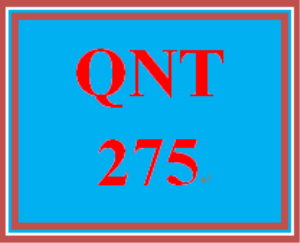# QNT 275 Week 4 Practice Set

PLDZ-3731
In Stock
\$ 6.00 USD
UopTutorialStore
Description

QNT 275 Week 4 Practice Set

https://uopcourses.com/category/qnt-275/

QNT 275 Week 4 Practice Set

Chapter 06, Section 6.1, Problem 013

Correct.

Find the area under the standard normal curve between z=-1.53 and z=2.37.

Round your answer to four decimal places.

A=

the tolerance is +/-2%

Chapter 06, Section 6.1, Problem 015a

Correct.

Obtain the area under the standard normal curve to the right of z=1.37.

Round your answer to four decimal places.

A=

the tolerance is +/-2%

Chapter 06, Section 6.2, Problem 019a

Correct.

Find the z value for x=33 for a normal distribution with μ=30 and σ=5.

Enter the exact answer.

z=

exact number, no tolerance

Chapter 06, Section 6.2, Intelligent Tutoring Problem 023

Compute probabilities.

Recall the following definitions from section 6.4 of the text.

The area under the normal curve from x = a to x = b with given mean and standard deviation is the probability that x assumes a value between x = a and x = b. If we are using Table IV in Appendix C, we need to standardize the random variable x using the formula z = (x− µ)/σ, before using the table.

Alternatively, you may use a graphing calculator to obtain more accurate calculations without standardizing the random variable x.

For example, using a TI83 plus we calculate the area under the normal curve from x = a to x = b by using the

normalcdf(a,b,µ,σ)

where µ is the mean and σ is the standard deviation of the normal distribution. We use 1E99 for ∞ and −1E99 for −∞, if needed.

Recent Reviews Write a Review
0 0 0 0 reviews